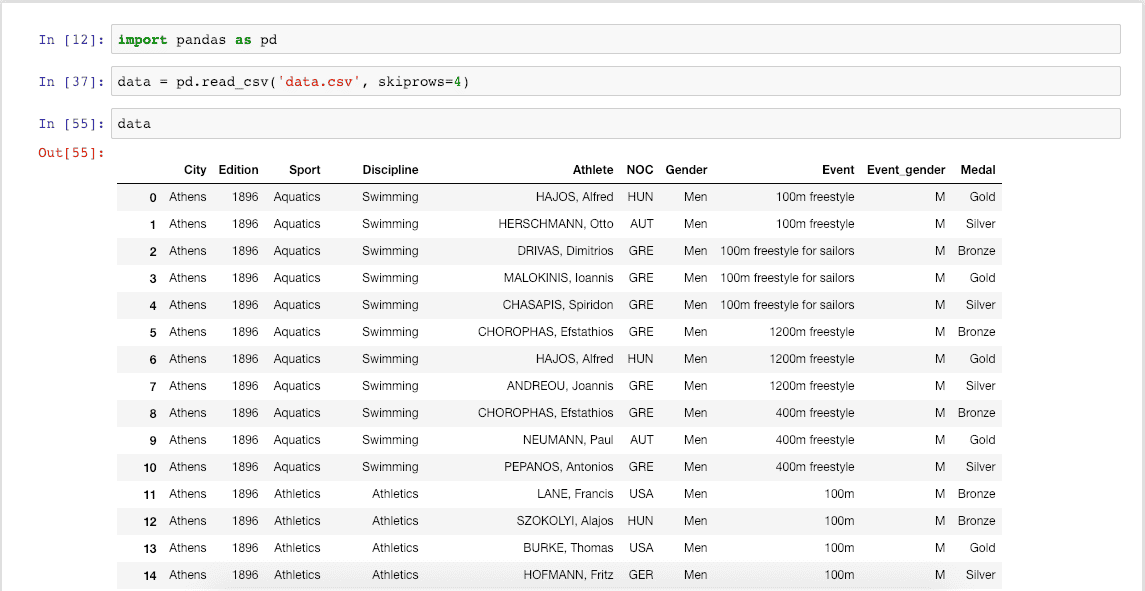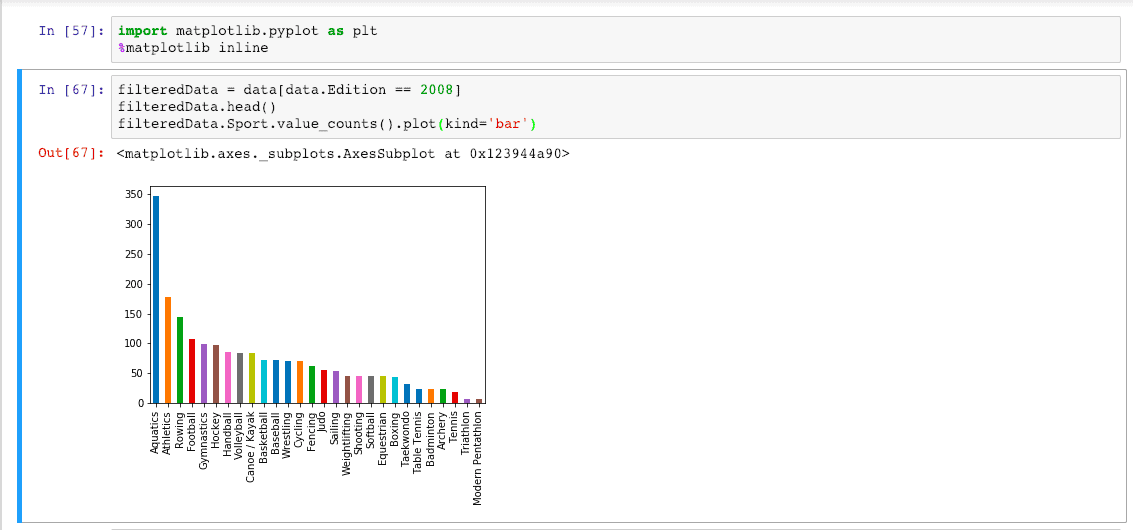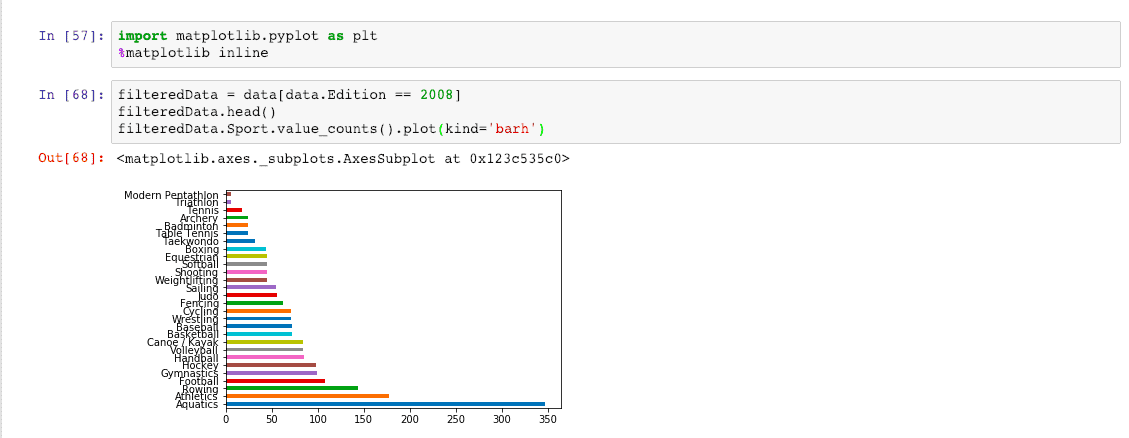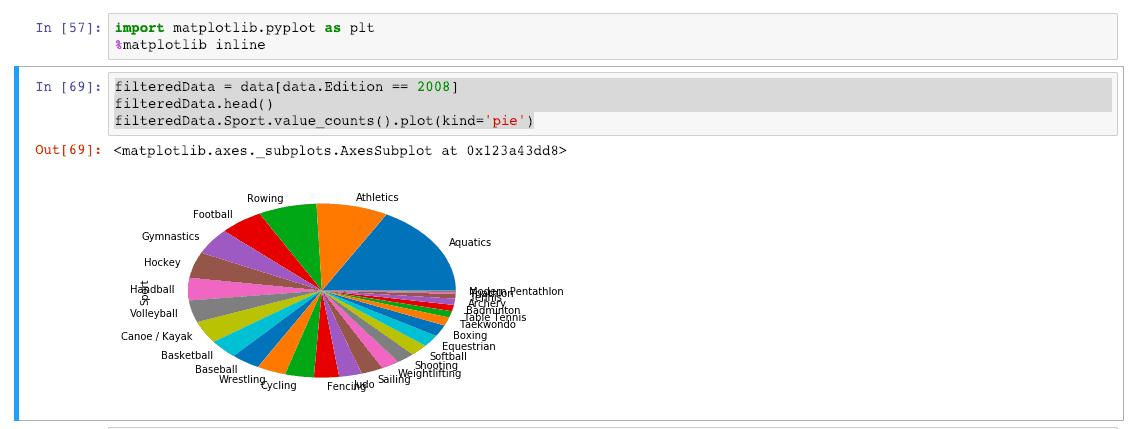# Matplotlib: A plotting Library for the Python

Python Matplotlib is a plotting library for the Python programming language and its numerical mathematics extension NumPy. In this tutorial, we will see how to use the Matplotlib library to report and chart using the matplotlib library.

## What is Matplotlib?

The matplotlib.pyplot is the collection of command styles and functions that make matplotlib work like MATLAB in Python.

Each pyplot function changes a figure, and we will be able to analyze the data based on that figure.

Matplotlib is the Python 2D plotting chart library that produces the publication quality figures in hardcopy and interactive environments.

Matplotlib can be used in the Python scripts, the Python and IPython shells, the Jupyter Notebook, web application servers, and four graphical user interface toolkits. Matplotlib is the basics of Python data visualization.

Matplotlib tries to make easy things as easy and hard things as possible. Thus, you can generate the plots, histograms, power spectra, bar charts, error charts, scatterplots, etc., with just a few lines of code.

So, in this demo, we will use Jupyter Notebooks to display different charts. Also, we need data to work on this project. So, you can save the CSV file from the below URL.

Now, open the Jupyter Notebook and import the Pandas Library first.

Write the following code inside the first cell in Jupyter Notebook.

`import pandas as pd`

Run the cell by hitting Ctrl + Enter.

Okay, now we will use the  read_csv() function of the DataFrame data structure in Pandas. So write the following code in the next cell.

```data = pd.read_csv('data.csv', skiprows=4)
data```

So, we have used the read_csv() function, skipped the first four rows, and then displayed the remaining ones. Run the cell and see the output. It will show the first 30 rows and the last 30 if so many exist. In our data file, there are above 29,000 rows. That is why we can see the first and last 30 rows.## Import Matplotlib

We can import the Matplotlib library using the following code. Next, write the following code inside the next Jupyter Notebook cell.

```import matplotlib.pyplot as plt
%matplotlib inline```

Now, hit the Ctrl + Enter, and it will import the library.

An iPython kernel works seamlessly with the Matplotlib.pyplot library.

You can see in the above code that we have used the %matplotlib inline magic command, which means that it will show different kinds of charts inside the Jupyter Notebook.

## Plot Types

There is a kind of Plot type which are following.

3. ##### plot(kind=pie): Best for reaching the parts of a whole system.

Now let’s take an example of a one-by-one chart in Jupyter Notebook.

Let’s plot a graph of different sports that takes part in the Olympics Edition 2008.

We have already imported the matplotlib.pyplot library in the Notebook, now we will use that to plot the graph of different sports.

## Plot a Line Chart using Matplotlib.pyplot Library

We will display the line chart. So let’s add the following code to the Jupyter Notebook.

```filteredData = data[data.Edition == 2008]
filteredData.Sport.value_counts().plot()```

Now, in the above code, first, we have the data for Olympics 2008 edition, and then we have to count the number of sports the Olympics has and plot the line graph based on that data. So the output of the above code in the Jupyter Notebook is the following.By default, the plot() function gives us the line chart.

## Plot a Bar Chart using Matplotlib.pyplot Library

We can also display the bar chart instead of the line chart. We must pass a parameter kind and value to the bar, showing the bar chart. See the following example. Write the following code in the cell.

```filteredData = data[data.Edition == 2008]
filteredData.Sport.value_counts().plot(kind='bar')```

Here, we have used the head() function to display the first five rows and plot the bar charts based on the sports count held in the 2008 Olympics.

The output is the following.The above bar chart is the Vertical Bar Chart.

We can also get the Horizontal plot using the following code.

`filteredData.Sport.value_counts().plot(kind='barh')`

We have passed the kind=’barh’ parameter, which will give us the following result.## Plot a Pie Chart using Matplotlib.pyplot Library

We can also display the pie chart instead of the bar chart. We must pass a parameter kind and value to the pie, showing the bar chart. See the following example. Write the following code in the cell.

```filteredData = data[data.Edition == 2008]